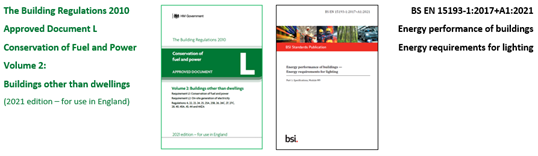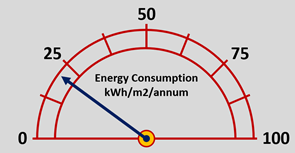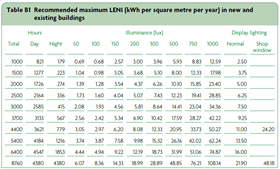## LIGHTING LEGISLATION – LENI

Further to the editorial written regarding the publication of the new Building Regulations 2010, Approved Document L on the Conservation of Fuel and Power, Volume 2 relating to buildings other than dwellings, where the information on system specific guidance for lighting, stated as one option, that general lighting should observe the Lighting Energy Numeric Indicator (LENI) method by following the details given within Appendix B, with the LENI method being an alternative approach for complying with the standards for lighting given in this approved document.

The LENI value has been around for many years and the background to the LENI calculation process comes from the European standard of EN 15193-1 on the energy performance of buildings for the energy requirements for lighting, published back in 2007, and the latest version of the implemented British Standard is BS EN 15193-1:2017+A1:2021, which specifies the calculated methodology for the evaluation of the energy performance of lighting systems in providing general illumination in residential and non-residential buildings, being applicable for both new and refurbished buildings.This method provides an alternative way of calculating the lighting energy performance of a building, rather than assessing the limits through the efficacy of each individual luminaire within the application and allows lighting designers greater flexibility in the design, allowing for the inclusion of several different types of luminaires, however the requirements of BS EN 15193-1 does not include any design requirements of the lighting system, of either the planning or specification of the lighting equipment installed within the application, whether that be for general illumination of for display lighting, alongside that of simulating any lighting scene control. The output of the calculations is to determine the Lighting Energy Numeric Indicator (LENI) value, which is based upon the total power consumption of the lighting load including parasitic load, measured over a duration period of one year and divided by the total area measured in square metres, excluding any non-illuminated areas. It shall be given in terms of kilowatt hours per square metre of the area per year (kW/m2/year).The standard BS EN 15193-1, offers three methods for the evaluation of the energy performance of lighting systems, being: Method 1, which provides the route for the calculation on the energy requirements for lighting in non-residential buildings where a comprehensive lighting system design has been performed and is suitable for new or refurbished buildings as well as for assessing existing buildings to provide the most accurate calculated LENI value. Method 2 is the quick calculation method and covers the calculation of the energy requirements of lighting systems for non-residential buildings where a comprehensive lighting system design has not been performed, making use of quick calculation and default data, with the result given as budget values. Method 3 covers the direct metering measurement of the energy being used by lighting system and can be used to verify the values obtained by the calculation methods.

Returning to the content of the Building Regulations, Approved Document L, Volume 2, where for determining the value of LENI, the calculation process is given as a series of steps, the details of which are discussed below.

1: To determine the lighting energy limit from the information contained within Table B1, shown below, based upon the annual hours of operation and the level of illuminance. If display lighting is used, the lighting energy limit for normal display lighting relates only to the areas or rooms in which display lighting is installed.2: To calculate the parasitic energy use (Ep), where, if the parasitic energy use is an unknown value, then an allowance of 0.3Watts/metre2 should be made for any control system, however if there is not a lighting control system being used, then the value of (Ep) is 0.

3: To determine the total power of lighting (Pl), which is the declared circuit power in Watts for all the luminaires within the space or area being calculated, when operating at full power, including LED driver power.

4: To determine the occupancy factor (Fo), where if there is no automatic control being used, then the value of (Fo) is 1, but if controls turn off the lights within a period of 20 minutes of the space or area having no occupancy, then (Fo) is 0.8.

5: To determine the factor for daylight (Fd), where if there is no daylight-linked dimming system being used, then the value of (Fd) is 1, but if the space or area has electric lighting which dims in response to daylight, then in those areas with adequate daylight, the (Fd) is 0.8. This may be taken as all areas within 6 metres of a window wall or in areas where 10% or more of the roof is translucent or made up of rooflights.

6: To determine the constant illuminance factor (Fc), where for systems that control the constant illuminance in this way have an (Fc) of 0.9, and those that do not have an (Fc) of 1.

Having now obtained the values for each of the factors to determine the Lighting Energy Numeric indicator, (LENI), now is the point at which the calculation process commences.

7: To calculate the daytime energy use (Ed), where the daytime energy use (Ed)is given as being the multiplication of the total installed lighting power (Pl), the occupancy factor (Fo), the daylight factor (Fd), the constant illuminance factor (Fc) and the daylight operating hours (Td), then divided by 1000.

8: To calculate the night-time energy use (En), where the night-time energy use (En) is given as being the multiplication of the total installed lighting power (Pl), the occupancy factor (Fo), the constant illuminance factor (Fc) and the night-time operating hours (Td), then divided by 1000.

9: To calculate the value of LENI, being the total energy (kWh) per square metre per year, where this is gained by the sum of the daytime energy, the night-time energy & the parasitic energy use per year divided by the area (A), as set out using the formula parasitic energy (Ep) x daylight energy (Ed) x night-time Energy (En) divided by the total area of the space or spaces being calculated.

The calculated LENI value should not exceed the lighting energy limit as specified in Table B1 for a given level of illuminance and the number of hours for which the system is operating.